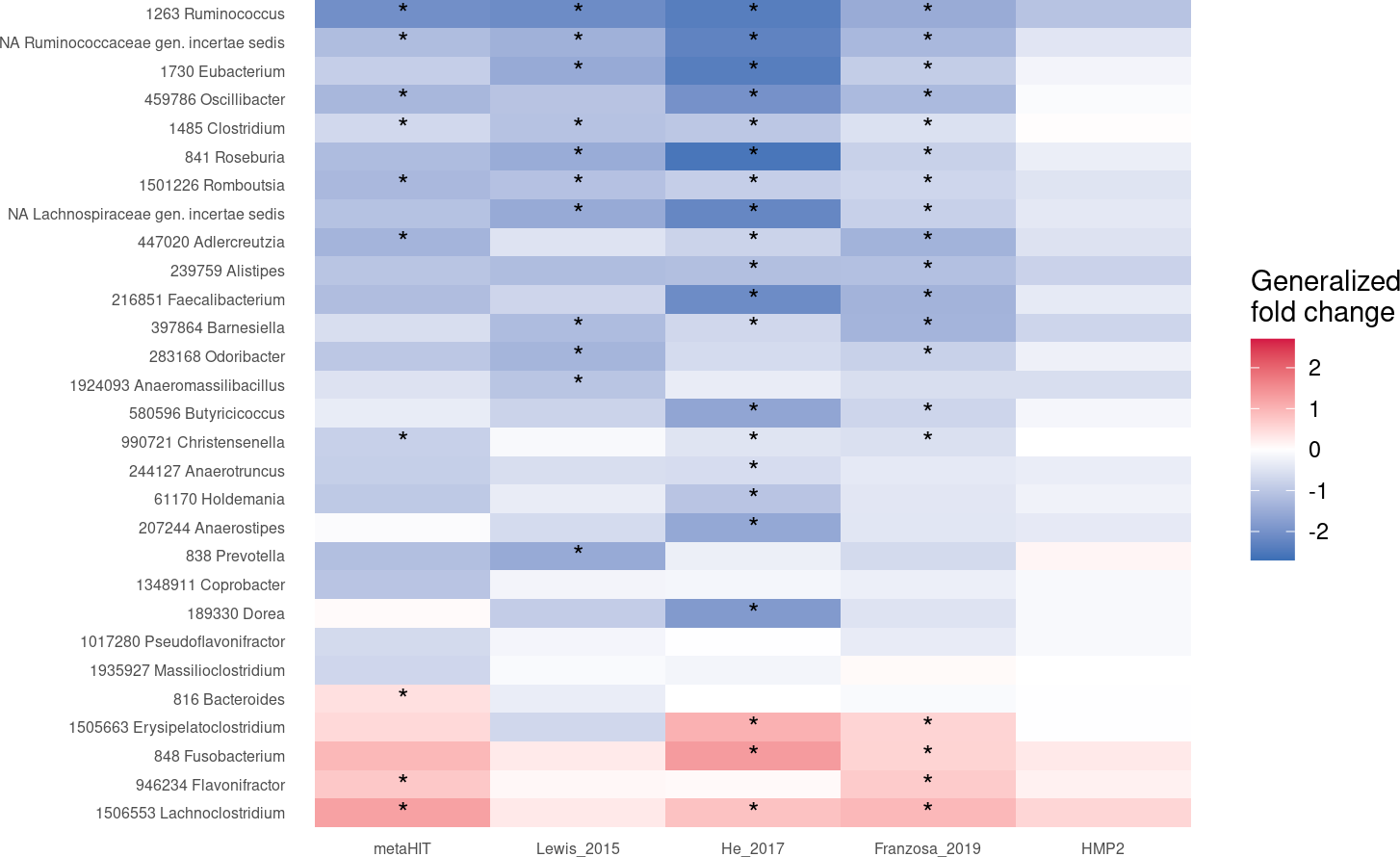# Contents

In this vignette, we want to demonstrate how `SIAMCAT` can facilitate metagenomic meta-analyses, focussing both on association testing and ML workflows. As an example, we use five different studies of Crohn’s disease (CD), since we have taxonomic profiles from five different metagenomic datasets available. Those studies are:

## 1.1 Setup

``````library("tidyverse")
library("SIAMCAT")``````

First, we load the data for all studies, which are available for download from the EMBL cluster. The raw data have been preprocessed and taxonomically profiled with mOTUs2 and were then aggregated at genus level.

``````# base url for data download
# datasets
datasets <- c('metaHIT', 'Lewis_2015', 'He_2017', 'Franzosa_2019', 'HMP2')
# features
check.names = FALSE, stringsAsFactors = FALSE, quote = '',
sep='\t')
feat <- as.matrix(feat)
# check that metadata and features agree
stopifnot(all(colnames(feat) == meta.all\$Sample_ID))``````

Let us have a look at the distribution of groups across the studies

``````table(meta.all\$Study, meta.all\$Group)
##
##                  CD CTR
##   Franzosa_2019  88  56
##   HMP2          583 357
##   He_2017        49  53
##   Lewis_2015    294  25
##   metaHIT        21  71``````

Some of the studies contain more than one sample for the same subject. For example, the HMP2 publication focussed on the longitudinal aspect of CD. Therefore. we want to take this into account when training and evaluating the machine learning model (see the vignette about Machine learning pitfalls) and when performing the association testing. Thus, it will be convenient to create a second metadata table containing a single entry for each individual.

``````meta.ind <- meta.all %>%
group_by(Individual_ID) %>%
filter(Timepoint==min(Timepoint)) %>%
ungroup()``````

# 2 Compare Associations

## 2.1 Compute Associations with SIAMCAT

To test for associations, we can encapsulate each dataset into a different `SIAMCAT` object and use the `check.associations` function:

``````assoc.list <- list()
for (d in datasets){

# filter metadata and convert to dataframe
meta.train <- meta.ind %>%
filter(Study==d) %>%
as.data.frame()
rownames(meta.train) <- meta.train\$Sample_ID

# create SIAMCAT object
sc.obj <- siamcat(feat=feat, meta=meta.train, label='Group', case='CD')
# test for associations
sc.obj <- check.associations(sc.obj, detect.lim = 1e-05,
feature.type = 'original',
fn.plot = paste0('./assoc_plot_',
d, '.pdf'))
# extract the associations and save them in the assoc.list
temp <- associations(sc.obj)
temp\$genus <- rownames(temp)
assoc.list[[d]] <- temp %>%
mutate(Study=d)
}
# combine all associations
df.assoc <- bind_rows(assoc.list)
df.assoc <- df.assoc %>% filter(genus!='unclassified')
##                                             genus fc auc p.adj   Study
## 159730 Thermovenabulum...1 159730 Thermovenabulum  0 0.5   NaN metaHIT
## 42447 Anaerobranca...2         42447 Anaerobranca  0 0.5   NaN metaHIT
## 1562 Desulfotomaculum...3   1562 Desulfotomaculum  0 0.5   NaN metaHIT
## 60919 Sanguibacter...4         60919 Sanguibacter  0 0.5   NaN metaHIT
## 357 Agrobacterium...5           357 Agrobacterium  0 0.5   NaN metaHIT
## 392332 Geoalkalibacter...6 392332 Geoalkalibacter  0 0.5   NaN metaHIT``````

## 2.2 Plot Heatmap for Interesting Genera

Now, we can compare the associations stored in the `df.assoc` tibble. For example, we can extract features which are very strongly associated with the label (single-feature AUROC > 0.75 or < 0.25) in at least one of the studies and plot the generalized fold change as heatmap.

``````genera.of.interest <- df.assoc %>%
group_by(genus) %>%
summarise(m=mean(auc), n.filt=any(auc < 0.25 | auc > 0.75),
.groups='keep') %>%
filter(n.filt) %>%
arrange(m)``````

After we extracted the genera, we plot them:

``````df.assoc %>%
# take only genera of interest
filter(genus %in% genera.of.interest\$genus) %>%
# convert to factor to enforce an ordering by mean AUC
mutate(genus=factor(genus, levels = rev(genera.of.interest\$genus))) %>%
# convert to factor to enforce ordering again
mutate(Study=factor(Study, levels = datasets)) %>%
# annotate the cells in the heatmap with stars
ggplot(aes(y=genus, x=Study, fill=fc)) +
geom_tile() +
scale_fill_gradient2(low = '#3B6FB6', high='#D41645', mid = 'white',
limits=c(-2.7, 2.7),
name='Generalized\nfold change') +
theme_minimal() +
geom_text(aes(label=l)) +
theme(panel.grid = element_blank()) +
xlab('') + ylab('') +
theme(axis.text = element_text(size=6))``````# 3 Study as Confounding Factor

Additionally, we can check how differences between studies might influence the variance of specific genera. To do so, we create a singel `SIAMCAT` object which holds the complete datasets and then we run the `check.confounder` function.

``````df.meta <- meta.ind %>%
as.data.frame()
rownames(df.meta) <- df.meta\$Sample_ID
sc.obj <- siamcat(feat=feat, meta=df.meta, label='Group', case='CD')
## + starting create.label
## Label used as case:
##    CD
## Label used as control:
##    CTR
## + finished create.label.from.metadata in 0.001 s
## + starting validate.data
## +++ checking overlap between labels and features
## + Keeping labels of 504 sample(s).
## +++ checking sample number per class
## +++ checking overlap between samples and metadata
## + finished validate.data in 0.049 s
check.confounders(sc.obj, fn.plot = './confounder_plot_cd_meta.pdf',
feature.type='original')
## Finished checking metadata for confounders, results plotted to: ./confounder_plot_cd_meta.pdf``````